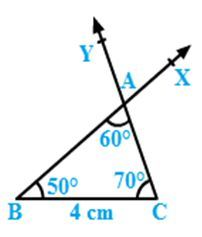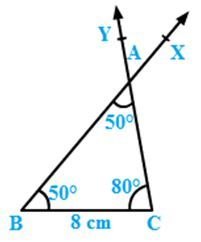×#### Thank you for registering.

One of our academic counsellors will contact you within 1 working day.

Click to Chat

1800-1023-196

+91-120-4616500

CART 0

• 0

MY CART (5)

Use Coupon: CART20 and get 20% off on all online Study Material

ITEM
DETAILS
MRP
DISCOUNT
FINAL PRICE
Total Price: Rs.

There are no items in this cart.
Continue Shopping• Complete JEE Main/Advanced Course and Test Series
• OFFERED PRICE: Rs. 15,900
• View Details

```Constructions Exercise 17.4

Question: 1

Construct ∆ABC in which BC = 4 cm, ∠B = 50° and ∠C = 70°.

Solution:Steps of construction:

Draw a line segment BC of length 4 cm.

Draw ∠CBX such that ∠CBX=50°.

Draw ∠BCY with Y on the same side of BC as X such that ∠BCY=70°.

Let CY and BX intersect at A.

ABC is the required triangle.

Question: 2

Draw ∆ABC in which BC = 8 cm, ∠B = 50° and ∠A = 50°.

∠ABC + ∠BCA + ∠CAB = 180°

∠BCA = 180° − ∠CAB − ∠ABC

∠BCA = 180°− 100° = 80°

Solution:Steps of construction:

Draw a line segment BC of length 8 cm.

Draw ∠CBX such that ∠CBX = 50°.

Draw ∠BCY with Y on the same side of BC as X such that ∠BCY = 80°.

Let CY and BX intersect at A.

Question: 3

Draw ∆ABC in which ∠Q = 80°, ∠R = 55° and QR = 4.5 cm. Draw the perpendicular bisector of side QR.

Solution:Steps of construction:

Draw a line segment QR = 4.5 cm.

Draw ∠RQX = 80° and ∠QRY = 55°.

Let QX and RY intersect at P so that PQR is the required triangle.

With Q as centre and radius more that 2.25 cm, draw arcs on either sides of QR.

With R as centre and radius more than 2.25 cm, draw arcs intersecting the previous arcs at M and N.

Join MN

MN is the required perpendicular bisector of QR.

Question: 4

Construct ∆ABC in which AB = 6.4 cm, ∠A = 45° and ∠B = 60°

Solution:Steps of construction:

Draw a line segment AB = 6.4 cm.

Draw ∠BAX = 45°.

Draw ∠ABY with Y on the same side of AB as X such that ∠ABY = 60°.

Let AX and BY intersect at C.

ABC is the required triangle.

Question: 5

Draw ∆ABC in which AC = 6 cm, ∠A = 90° and ∠B = 60°.

∠A + ∠B + ∠C = 180°

Therefore ∠C = 180°− 60°− 90°= 30°

Solution:Steps of construction:

Draw a line segment AC = 6 cm.

Draw ∠ACX = 30°.

Draw ∠CAY with Y on the same side of AC as X such that ∠CAY = 90°.

Join CX and AY. Let these intersect at B.

ABC is the required triangle where angle ∠ABC = 60°.
```### Course Features

• 728 Video Lectures
• Revision Notes
• Previous Year Papers
• Mind Map
• Study Planner
• NCERT Solutions
• Discussion Forum
• Test paper with Video Solution